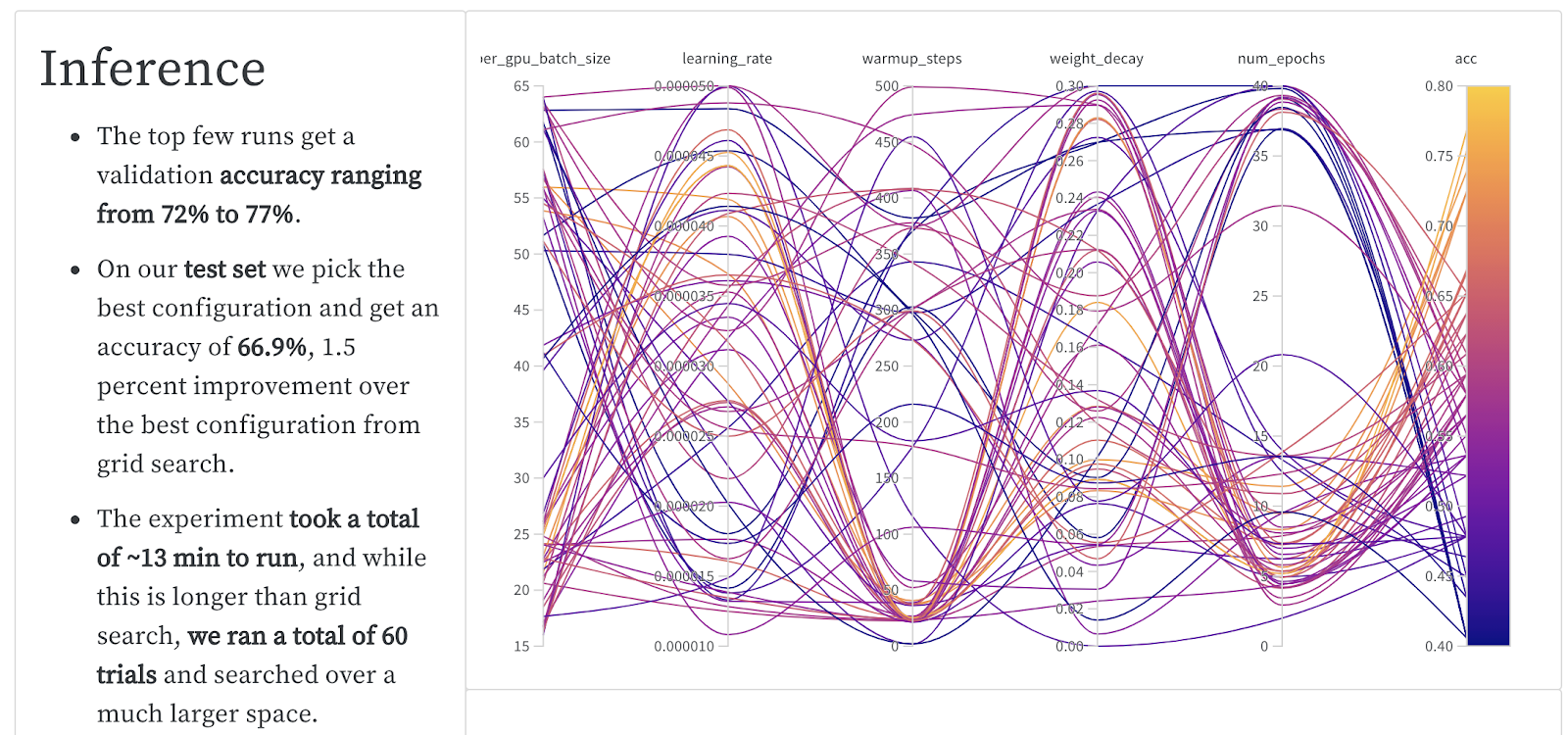# Hyperparameter Search with Transformers and Ray Tune

##### A guest blog post by Richard Liaw from the Anyscale team

With cutting edge research implementations, thousands of trained models easily accessible, the Hugging Face transformers library has become critical to the success and growth of natural language processing today.

For any machine learning model to achieve good performance, users often need to implement some form of parameter tuning. Yet, nearly everyone (1, 2) either ends up disregarding hyperparameter tuning or opting to do a simplistic grid search with a small search space.

However, simple experiments are able to show the benefit of using an advanced tuning technique. Below is a recent experiment run on a BERT model from Hugging Face transformers on the RTE dataset. Genetic optimization techniques like PBT can provide large performance improvements compared to standard hyperparameter optimization techniques.

 Algorithm Best Val Acc. Best Test Acc. Total GPU min Total $cost Grid Search 74% 65.4% 45 min$2.30 Bayesian Optimization +Early Stop 77% 66.9% 104 min $5.30 Population-based Training 78% 70.5% 48 min$2.45

If you’re leveraging Transformers, you’ll want to have a way to easily access powerful hyperparameter tuning solutions without giving up the customizability of the Transformers framework.In the Transformers 3.1 release, Hugging Face Transformers and Ray Tune teamed up to provide a simple yet powerful integration. Ray Tune is a popular Python library for hyperparameter tuning that provides many state-of-the-art algorithms out of the box, along with integrations with the best-of-class tooling, such as Weights and Biases and tensorboard.

To demonstrate this new Hugging Face + Ray Tune integration, we leverage the Hugging Face Datasets library to fine tune BERT on MRPC.

To run this example, please first run:

pip install "ray[tune]" transformers datasets scipy sklearn torch

Simply plug in one of Ray’s standard tuning algorithms by just adding a few lines of code.

from datasets import load_dataset, load_metric
from transformers import (AutoModelForSequenceClassification, AutoTokenizer,
Trainer, TrainingArguments)

tokenizer = AutoTokenizer.from_pretrained('distilbert-base-uncased')

def encode(examples):
outputs = tokenizer(
examples['sentence1'], examples['sentence2'], truncation=True)
return outputs

encoded_dataset = dataset.map(encode, batched=True)

def model_init():
return AutoModelForSequenceClassification.from_pretrained(
'distilbert-base-uncased', return_dict=True)

def compute_metrics(eval_pred):
predictions, labels = eval_pred
predictions = predictions.argmax(axis=-1)
return metric.compute(predictions=predictions, references=labels)

# Evaluate during training and a bit more often
# than the default to be able to prune bad trials early.
# Disabling tqdm is a matter of preference.
training_args = TrainingArguments(
"test", evaluation_strategy="steps", eval_steps=500, disable_tqdm=True)
trainer = Trainer(
args=training_args,
tokenizer=tokenizer,
train_dataset=encoded_dataset["train"],
eval_dataset=encoded_dataset["validation"],
model_init=model_init,
compute_metrics=compute_metrics,
)

# Default objective is the sum of all metrics
# when metrics are provided, so we have to maximize it.
trainer.hyperparameter_search(
direction="maximize",
backend="ray",
n_trials=10 # number of trials
)


By default, each trial will utilize 1 CPU, and optionally 1 GPU if available. You can leverage multiple GPUs for a parallel hyperparameter search by passing in a resources_per_trial argument.

You can also easily swap different parameter tuning algorithms such as HyperBand, Bayesian Optimization, Population-Based Training:

To run this example, first run: pip install hyperopt

from ray.tune.suggest.hyperopt import HyperOptSearch
from ray.tune.schedulers import ASHAScheduler

trainer = Trainer(
args=training_args,
tokenizer=tokenizer,
train_dataset=encoded_dataset["train"],
eval_dataset=encoded_dataset["validation"],
model_init=model_init,
compute_metrics=compute_metrics,
)

best_trial = trainer.hyperparameter_search(
direction="maximize",
backend="ray",
# Choose among many libraries:
# https://docs.ray.io/en/latest/tune/api_docs/suggestion.html
search_alg=HyperOptSearch(metric="objective", mode="max"),
# Choose among schedulers:
# https://docs.ray.io/en/latest/tune/api_docs/schedulers.html
scheduler=ASHAScheduler(metric="objective", mode="max"))


It also works with Weights and Biases out of the box!### Try it out today:

If you liked this blog post, be sure to check out: